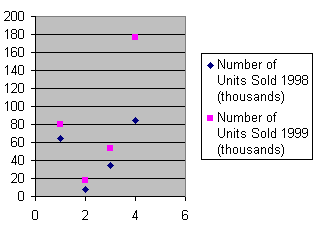# scatter diagram

(redirected from scatter diagrams)
Also found in: Dictionary, Medical.
Related to scatter diagrams: correlation

## scatter diagram

(petrology)
(statistics)
A plot of the pairs of values of two variates in rectangular coordinates. Also known as scatter gram.

or

## scattergram

a diagram produced on a graph to illustrate the relationship between two variables by indicating the values of these for each case in the sample. It may be a first stage in data analysis, allowing ‘eye-balling’ of the data to observe any association or CORRELATION between the variables.

## scatter diagram

A graph plotted with dots or some other symbol at each data point. Also called a "scatter plot" or "dot chart."

A Scatter DiagramReferences in periodicals archive ?
Scatter diagram of the normalized scattering vectors in standard 2simplex is shown in Fig.
Scatter diagrams corresponding to different cross sections of the abdomen were shown in Figure 8(a) and (b).
The scatter diagrams for observed and modelled waves are similar at all observation sites for low and moderate wave conditions up to wave heights of 3 m (Fig.
They include but are not limited to scatter diagrams, Pareto charts, histograms, regression analyses and fishbone diagrams.
Scatter diagrams plot the occurrence(s) of failure, or deviations from the norm enabling confirmation or denial of a suspected relationship, between, for example, supply and production, or production and delivery.
Scatter diagrams help us look for a cause and effect relationship, as in the amount of a drug given and the subsequent pain rating.
Variables Data * Important Aspects of Data Collection * Diagrams for Problem Solving * Pareto Diagrams * Histograms * Scatter Diagrams and Correlation Tables * Recording Data * Sampling
Graphics Server includes a dynamic charting application that takes user input and creates and modifies various charts, such as pie charts, bar charts, and scatter diagrams.
The most useful formats have withstood the test of time: Pie charts, horizontal bar charts, vertical bar or horizontal line charts, vertical column or histogram frequency distribution charts and scatter diagrams have become classics because they work.
This article outlines the basic procedure for creating scatter diagrams, illustrates various practical applications for graphic analysis in the sales comparison approach to value, and identifies possible pitfalls.
We can use scatter diagrams to display the correlation between the random variables X and Y graphically.

Site: Follow: Share:
Open / Close